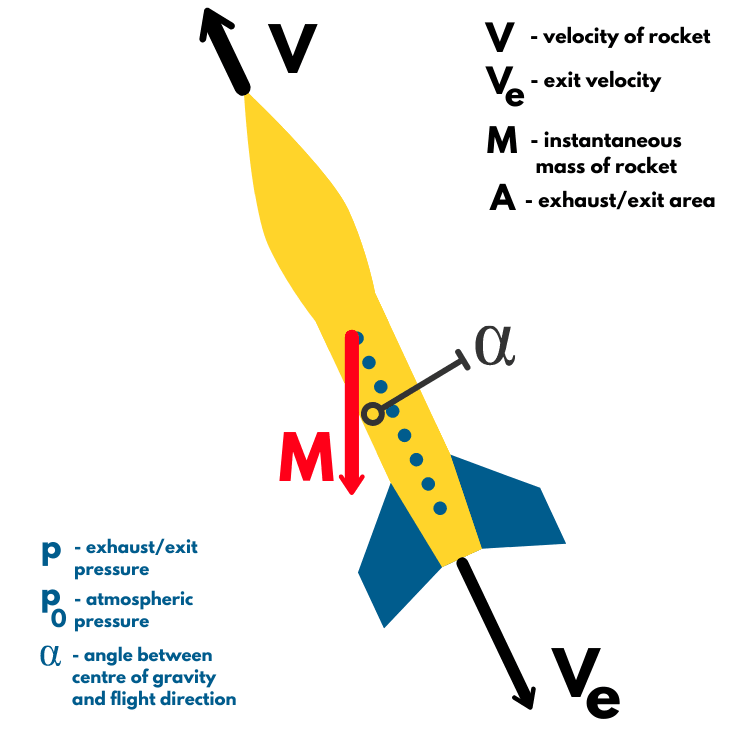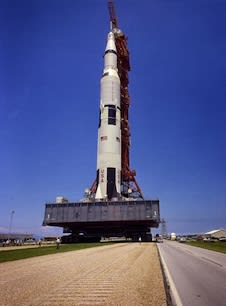# The Rocket Equation - Sutton Program Article 3

This article will accompany the third video in our series made possible by RS Components Grass Roots aiming to introduce key rocketry concepts and principles. This article will explore the rocket equation, also known as the ideal or classical rocket equation, and show why it is one of the most important equations in modern rocketry. Written by Sean Clark and Kieran Webb

### Introduction

The Tsiolkovsky rocket equation, otherwise known as the ideal rocket equation or classical rocket equation, was derived by a famous and pioneering scientist by the name of Konstantin Tsiolkovsky. During his life, he made many positive contributions to the fields of rocketry and astronautics. Arguably his most outstanding discovery, this equation is one of the most important principles in rocketry and still essential to the field today.### A breakdown of the variables

V = Veq * ln(M0/Mf)

Shown above is one form of the equation. The variables are as follows.

• ∆V: pronounced as ‘delta V’, this is the total change in velocity of the rocket, also equal to the rocket system’s impulse per unit mass – the impulse on a body is equal to the average force applied across the system over time. In the case of a rocket, the force referred to is the thrust
• Veq: the effective/equivalent exhaust velocity
• ln: the natural logarithm, a mathematical function
• Mo: the mass of the entire rocket system including propellant (i.e., the wet mass)
• Mf: the mass of the rocket system excluding propellant (i.e., the dry mass)

### The derivation & explanation

In the case of this example, we will choose to neglect the aerodynamic forces (lift and drag).

We will consider the rocket below in powered flight. Shown on the diagram are the variables we’ll need to take into consideration.The velocity of the rocket ‘V’ describes the velocity of the rocket- we’ll need to think of this separately from the exhaust velocity ‘e’ acting in the opposite direction.

The instantaneous mass of the rocket ‘M’ is equal to the total mass of the rocket at a given time.  Our exhaust/exit area ‘A’ refers to the nozzle area; our exhaust/exit pressure ‘p0 refers to the pressure of the exhausted fuel and ‘p0refers to the atmospheric pressure. α’ (alpha) is the angle between the centre of gravity and the direction of flight.

Keep in mind that these variables will all change constantly during a powered flight.

A good way to visualise a rocket’s constant change in mass due to producing thrust during powered flight is to imagine throwing small amounts of mass overboard at a constant rate – this will be written as ‘dm’, as it is comparatively very small compared to the total mass of the system.

dM’ is the rate of change of the instantaneous rocket mass which is also equal to the change in mass flow rate over time, ‘ṁ*dt’. However, as the instantaneous mass ‘M’ decreases as a result of the exhausted mass dm, the sign on the right-hand side of the equation is negative. To summarise:

dm = ṁ*dt = -dM

In the previous article in our program, we introduced the concept of the conservation of momentum and its importance within rocketry. This principle acts as our starting point for our equation. First, we must find the total change in momentum across the whole system. This means we will consider the larger rocket mass and the smaller exhausted mass.

For the larger rocket mass: M*(V+dV) – M*V = M*dV

For the smaller exhausted mass: dm*(V-Ve) – dm*V = -dm*Ve

The total momentum change across the whole system is the summation of these two separate momenta, which is equal to:

M*dV – dm*Ve

We must now consider the forces acting upon the system, keeping in mind that we are not considering the aerodynamic forces as it makes things considerably more complicated. The main forces we are concerned with here are the pressure force and the gravitational force.

The pressure force acts in the same direction as V, and is equal to the change between atmospheric pressure and the pressure at the exhaust, multiplied by the nozzle area:

(p-p0)*A

The gravitational force acts at angle Alpha, and is equal to the instantaneous mass multiplied by the gravitational constant:

M*g*cos(α)

As before, we can combine these two to get the total force acting upon the system:

(p-p0)*A – M*g*cos(α)

Here we can apply the concept of impulse. As mentioned previously, this is equal to the change in momentum of the system. In other words, it is the change in the total force applied to the system over time, so all we need to do is multiply the terms above by dt. We have already derived the total change in momentum, which we can now set equal to the above equation:

M*dV – dm*Ve = (p-p0)*A – M*g*cos(α)

We can ignore the gravitational force M*g*cos(α) and rearrange the equation:

M*dV = [(p-p0)*A] * dt + dmVe

Remembering that dm = ṁ*dt , we can substitute these terms into the equation:

M*dV = [(p-p0)*A] * dt + ṁ*dt *Ve

Which can be simplified to:

M*dV = [(p-p0)*A + ṁ*Ve ] * dt

Here we can introduce a very useful relationship which tidies up this equation quite a bit. The equivalent exhaust velocity ‘Veq’ is also equal to the exhaust velocity plus total force on the system divided by the mass flow rate:

Veq = Ve + [(p-p0)*A]/ ṁ

Substituting this into our equation, we get:

M*dV = Veq * ṁ * dt

Remembering that ṁ*dt = -dM :

M*dV = -Veq * dM

Rearranging the equation for dV:

dV = -Veq * dM/M

We can now integrate with respect to time, noting that the boundaries of the integral are the wet mass of the rocket and the dry mass of the rocket.

V = -Veq * [ln(Mf) – ln(M0)]

Rearranging the equation to remove the double negative signs:

V = Veq * [ln(M0) – ln(Mf)]

Applying some algebra to the natural logs, we can make a ratio:

V = Veq * ln(M0/Mf)

This is the full derivation of the equation from the video above. As a side note, M0/Mfcan simply be abbreviated as ‘MR’, or mass ratio. Another form of this equation relates to specific impulse, ‘Isp’, which is merely the impulse per unit mass, as the specific impulse multiplied by the standard gravity g0 is equal to the equivalent exhaust velocity- this is useful in case we want to calculate the specific impulse required for a given delta V.

DeltaV = Veq * ln(MR) = Isp * g0* ln(MR)

### Applications of delta V

One of the most important considerations that has to be made when it comes to space exploration is the change in velocity. This is because different areas of space require a different delta V. Once the initial and final masses and the exhaust velocity of the vehicle are known, the rocket equation can be used to calculate the total change in velocity.In order to achieve orbit, an object must complete an entire revolution about a planet ( this could be a planet around a star). Different orbital heights require different accelerations as the gravitational force pulling the object towards the surface is greater as distance lessens, a greater force is needed to withstand this pull. To complete a low earth orbit, delta V must be around 9,400ms-1 – this is fast enough to get from Glasgow to London in just over a minute!

### Got Fuel?

The obvious answer to a lot of rocket questions is to simply carry more fuel as more fuel means longer propulsion time which means they can fly for longer and travel further but unfortunately it is not quite as simple.

Imagine 2 rockets, both with the same propulsion system (and same force production), but one has been lengthened so it can carry more fuel. The extra mass from the fuel will add a considerable amount of weight but so will the extra materials that are needed to contain it as well.

This adds up to a very large initial mass increase – looking back at Newton’s equation; F = m*a, this means that the same force will produce a proportionally lower acceleration. If the rocket becomes too heavy it won’t even be able to achieve take-off. This is why staging rockets is so important and also why it is impossible with today’s technology for a rocket to get into orbit with only a single stage.

### Staging & the Saturn V rocket

Staging has been introduced previously within the program in our History of Rocketry article. A multi-stage rocket consists of several modules, each with their own independent propulsion systems. Once a stage has exhausted its fuel, it detaches from the body of the rocket, reducing the total mass. Because of this, the delta V must be calculated for each section. Rockets can be designed depending on the required velocity change necessary to achieve the desired range.

Staging was used in the famous Saturn V rocket used to take American astronauts to the moon in 1969. This rocket can be split into three main stages: section 1 – S-IC, section 2 – S-II and section 3 – S-IVB. Each associated delta V is analysed individually and then added together to give the total delta V for the entire rocket.Section 1 had the most difficult job of take-off with the greatest mass of the rocket- this is why the first stage has the most powerful engines and is heaviest (2,300,000 kg wet weight but only 131,000 kg). The delta V of section 1 is 3500ms-1.

Section 2 was considerably lighter and was responsible for thrusting the rocket through the upper atmosphere with a delta V of 5500ms-1 (dry weight: 36,000 kg, wet weight: 480,000 kg).

The third and final section provided the thrust to get the astronauts to the moon. This section is the lightest with 109,000 kg of fuel added to a dry weight of just 10,000 kg. The delta V of this section is 8900ms-1. Once delta V is known for all stages it is simply added together for the total delta V of the vehicle – this comes out to be 17,900 ms-1.

### Conclusion

This is the process used by rocket engineers all across the world to calculate the theoretical range of any modern rocket with respect to its velocity change, as long as the size of its payload and exhaust velocity is also known.

With the knowledge that you’ve gained from the program so far, you should understand some of the most basic and essential principles used in rocketry- calculations that must be made before an engineer can even think about getting their rocket off the ground.

In our next article, we will cover rocket propulsion types and techniques. Thanks for reading, and happy learning!

Parts in this series:We are a University of Glasgow based engineering project, focused on launching Scotland's first student-lead, high-powered rocket.